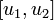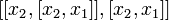# Basic Lie product

(Redirected from Basic commutator)

## Definition

The term basic Lie product is used in the following context. Let$A$ be the free associative algebra over the field of rational numbers$\mathbb{Q}$ using$n$ generators$x_1,x_2,\dots,x_n$. In other words,$A = \mathbb{Q} \langle x_1,x_2,\dots,x_n \rangle$.

The term basic Lie product is used for some of the iterated Lie products involving the variables$x_i$. There are two versions of the definition, one relying on left-normed commutators, and the other relying on right-normed commutators. Note that the basic commutators for the left-normed definition are not, in general, left-normed commutators. Similarly for the right-normed case.

### Left-normed definition

The definition has a number of preliminaries, which we first attend to:

Term Meaning
lexicographic ordering on associative monomials We define it as follows:$x_{i_1}x_{i_2} \dots x_{i_m} < x_{j_1}x_{j_2} \dots x_{j_n}$ either if, for some$k$, we have$i_1 = j_1, i_2 = j_2, \dots i_{k-1} = j_{k-1}$ and$i_k < j_k$, or if the right side is an initial segment of the left side.
regular word (also called Lyndon word) A word that is strictly greater than all its cyclic permutations. A word$u$ is regular if, whenever$u = vw$ for non-empty words$v,w$, then$u > wv$.
weight of a Lie product the number of monomial terms that appear in it. Includes repetition counts. Thus, the weight of$[[x_1,x_2],[x_1,x_3]]$ is 4.
underlying associative monomial of a Lie product the monomial word obtained by dropping all the Lie brackets and reading the word as an associative word. Note that this is not the same as the element of$A$ that one would get by expanding the Lie brackets. However, it is one of the monomials that occurs in that word. For instance, the underlying associative monomial of$[[x_1,x_2],x_3]$ is$x_1x_2x_3$, whereas the polynomial for the Lie product itself is$x_1x_2x_3 - x_2x_1x_3 - x_3x_1x_2 + x_3x_2x_1$.

Consider a Lie product$w$ involving the generators$x_i$. It is termed a basic Lie product if either it has weight one or it satisfies all of the following conditions, after we first note$w = [u,v]$ for smaller Lie products$u,v$:

1. Both$u$ and$v$ are basic Lie products.
2. The underlying associative monomial of$u$ is greater than the underlying associative monomial of$v$ in the lexicographic ordering (see above).
3. If$u$ has weight more than one, note it of the form$[u_1,u_2]$, and then it must be true that the underlying associative monomial of$u_2$ is$\le$ the underlying associative monomial of$v$. (Note: If$u$ has weight one, this condition need not be checked).

The following basic facts are true:

• The underlying associative monomial of a basic Lie product is a regular word.
• For every regular word, there is a unique basic Lie product having that as the underlying associative monomial.

## Facts

Consider the free$\mathbb{Q}$-Lie algebra on$x_1,x_2,\dots,x_n$. The basic Lie products defined here form a basis for this Lie algebra as a$\mathbb{Q}$-vector space. Moreover, the basic Lie products of length$r$ form a basis for the degree$r$ homogeneous component of the free Lie algebra.

This basis is termed the Lyndon basis or the Shirshov basis.

In fact, the basic Lie products form a free generating set for the degree$r$ homogeneous component of the$\mathbb{Z}$-Lie algebra, which is a free abelian group.

This fact can also be used to give a formula for the dimension of the homogeneous graded components of free Lie algebras. For more, see formula for dimension of graded component of free Lie algebra.

## Particular cases

### Basic Lie products in two variables: left-normed version

The variables are taken as$x_1$ and$x_2$. We list all the basic Lie products in the left-normed version of weight up to five.

Basic Lie product Weight Underlying associative monomial (regular word) Lie product as associative polynomial$x_1$ 1$x_1$$x_1$$x_2$ 1$x_2$$x_2$$[x_2,x_1]$ 2$x_2x_1$$x_2x_1 - x_1x_2$$[[x_2,x_1],x_1]$ 3$x_2x_1^2$$x_2x_1^2 - 2x_1x_2x_1 + x_1^2x_2$$[x_2,[x_2,x_1]]$ 3$x_2^2x_1$$x_2^2x_1 - 2x_2x_1x_2 + x_1x_2^2$$[[[x_2,x_1],x_1],x_1]$ 4$x_2x_1^3$$x_2x_1^3 - 3x_1x_2x_1^2 + 3x_1^2x_2x_1 - x_1^3x_2$$[x_2,[[x_2,x_1],x_1]]$ 4$x_2^2x_1^2$$x_2^2x_1^2 - 2x_2x_1x_2x_1 + x_2x_1^2x_2 - x_2x_1^2x_2 + 2x_1x_2x_1x_2 + x_1^2x_2^2$$[x_2,[x_2,[x_2,x_1]]]$ 4$x_2^3x_1$ PLACEHOLDER FOR INFORMATION TO BE FILLED IN: [SHOW MORE]$[[[[x_2,x_1],x_1],x_1],x_1]$ 5$x_2x_1^4$ PLACEHOLDER FOR INFORMATION TO BE FILLED IN: [SHOW MORE]$[[x_2,x_1],[[x_2,x_1],x_1]]$ 5$x_2x_1x_2x_1^2$ PLACEHOLDER FOR INFORMATION TO BE FILLED IN: [SHOW MORE]$[x_2,[[[x_2,x_1],x_1],x_1]]$ 5$x_2^2x_1^3$ PLACEHOLDER FOR INFORMATION TO BE FILLED IN: [SHOW MORE]$[[x_2,[x_2,x_1]],[x_2,x_1]]$ 5$x_2^2x_1x_2x_1$ PLACEHOLDER FOR INFORMATION TO BE FILLED IN: [SHOW MORE]$[x_2,[x_2,[[x_2,x_1],x_1]]]$ 5$x_2^3x_1^2$ PLACEHOLDER FOR INFORMATION TO BE FILLED IN: [SHOW MORE]$[x_2,[x_2,[x_2,[x_2,x_1]]]]$ 5$x_2^4x_1$ PLACEHOLDER FOR INFORMATION TO BE FILLED IN: [SHOW MORE]